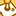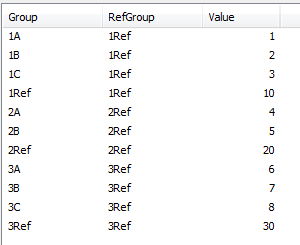# QlikView App Dev

Discussion Board for collaboration related to QlikView App Development.

Announcements
Customer & Partners, DEC. 9, 11 AM ET: Qlik Product & Strategy Roadmap Session: Data Analytics REGISTER NOW
cancel
Showing results for
Did you mean:Contributor II

## Set Analysis to aggregate on calculated (or associated) dimension value

I need to create a straight table where the aggregations in one column must be based on a specific/associated dimension, but it's no the dimension 'at that level' in the table.   I've created a simple project to illustrate (attached).

For example, consider the following data.  Notice we have a Group field by which we want to aggregate the Value field.  Each Group has a specific group that we'll want to aggregate in the same row.  So for Groups 1A, 1B, 1C... the RefGroup (reference group) group is 1Ref.In the table below, we have the desired output.  So we see the two dimensions (Group and RefGroup).  Our first metric (Sum) is just an normal aggregation on the Group.  The 2nd metric (Ref Sum) is an aggregation for the RefGroup associated with that Group.  So for 1A, 1B, and 1C, it's going to be an aggregation of Group = 1Ref.In sample data above, the *Ref values are always larger than the other values, but this is fake data, the actual values will vary.   And in the final output, we'll exclude the 'Ref' dimensions from Group, so they will not appear.  So we'll see rows for 1A, 1B, 1C, 2A, 2B, 3A, 3B, and 3C.

The question is how to create that 2nd metric above (Ref Sum) so that is aggregates just the value where essentially Group=RefGroup.

I've tried varying Set Analysis solutions, but since Set Analysis is not evaluated row-by-row, perhaps it's not possible?  I'm hitting a wall on this, and hoping someone can help.  Thanks so much for any ideas or insights.

NOTE:  If this is not possible via an expression, by plan B is to pre-aggregate values in the model as another table. But before going that route, I'd prefer to create an expression in the chart if possible.

Labels (2)

• ### straight table

1 Solution

Accepted SolutionsMVP

or just expression approach

=sum(total <RefGroup> aggr(sum({<Group={"=Group=RefGroup"}>} Value),Group))

9 RepliesSpecialist

Are you precluded from calculating Ref Sum in the script?

Finding the maximum of Value grouped by RefGroup gets what you display as desired output.Contributor II
Author

Thanks for the reply.  No, that's certainly an option.  So I could just create another table with the aggregates (sums) grouped by RefGroup (where Group=RefGroup).  For example:

TableRef:
Resident Table1
where Group = RefGroup
group by RefGroup;

Looks like this is indeed what I'll have to do. But I posted here just because this seems like something that should be possible at the record-level in a chart, using Set Analysis perhaps.  So I just wanted to get the input of folks here to see if I was missing something.Specialist

It doesn't appear you are using the sum function though, but you certainly could. More like the max value. I used;

Table1:
Group, RefGroup, Value
1A, 1Ref, 1
1B, 1Ref, 2
1C, 1Ref, 3
1Ref, 1Ref, 10
2A, 2Ref, 4
2B, 2Ref, 5
2Ref, 2Ref, 20
3A, 3Ref, 6
3B, 3Ref, 7
3C, 3Ref, 8
3Ref, 3Ref, 30
];

/* Get max */

MaxRefValue:
Left Join(Table1)
Max(Value) as RefMax,
RefGroup
Resident Table1
Group By RefGroup;

/* Get sum */

SumRefValue:
Left Join(Table1)
Sum(Value) as RefSum,
RefGroup
Resident Table1
Where Not WildMatch(Group,'*ref*')
Group By RefGroup;

If you desire the record level within a table approach I'm sure someone here can do it. 😉

HTH,

JohnContributor II
Author

Thanks again John.

I'm using the SUM in the way I did because for all records where RefGroup="1Ref", I need to get the sum where RefGroup="1Ref" AND Group="1Ref".  So I don't want to sum all the 1Ref records, and I don't want the max() cause it won't always be the max.

So all those that are 1A, 1B, and 1C will be 10, because that's the value of the RefGroup ("1Ref") for those records.  But it's just 10 in my fake data here. It could be that the 1Ref/1Ref value is 2, for example.

All good suggestions though, and helpful feedback.  Thank you again!MVP

Data:
Group, RefGroup, Value
1A, 1Ref, 1
1B, 1Ref, 2
1C, 1Ref, 3
1Ref, 1Ref, 10
2A, 2Ref, 4
2B, 2Ref, 5
2Ref, 2Ref, 20
3A, 3Ref, 6
3B, 3Ref, 7
3C, 3Ref, 8
3Ref, 3Ref, 30 ];

Left Join(Data)
sum(Value) as [Ref Sum]
Resident Data
where Group=RefGroup
Group by RefGroup;

Now, simply add Sum([Ref Sum]) expression in chartMVP

alternative, Flag method approach

Data:
LOAD *, if(Group=RefGroup,1,0) as Flag INLINE [
Group, RefGroup, Value
1A, 1Ref, 1
1B, 1Ref, 2
1C, 1Ref, 3
1Ref, 1Ref, 10
2A, 2Ref, 4
2B, 2Ref, 5
2Ref, 2Ref, 20
3A, 3Ref, 6
3B, 3Ref, 7
3C, 3Ref, 8
3Ref, 3Ref, 30 ];

Ref Sum expression: =sum(total <RefGroup> aggr(sum({<Flag={1}>} Value),Group))MVP

or just expression approach

=sum(total <RefGroup> aggr(sum({<Group={"=Group=RefGroup"}>} Value),Group))Contributor II
Author

Thanks so much!

I like the ideas offered here on both using a Flag in the model or just JOIN'ing to add the aggregation to the model.

But doing with straight SET ANALYSIS was the initial objective, and you've offered just that solution. I had danced around it, but I was neglecting to use TOTAL <RefGroup> to limit dimensions for the AGGR().MVP